# How to Concatenate Cells and Add Different Separators in Excel?

Sometimes we want to concatenate words which are separated into different cells into one big column, how can we do? Now we will share you some small tips to concatenate words in Excel, and you can also add different separators between words if you like.

## Concatenate cells by CONCATENATE Function

This function is very useful for concatenating words. Here are some samples to demonstrate this function.

a. Insert space between words by CONCATENATE FUNCTION.

Space is a quite common separator for concatenate words. See below table, we have two columns, and Hello word is listed in column A->A1, world in column B->B1; Good in column A->A2, Evening in column B->B2.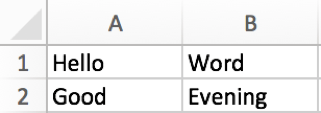Step 1: In cell C1, enter the formula =CONCATENATE(A1,” “,B1).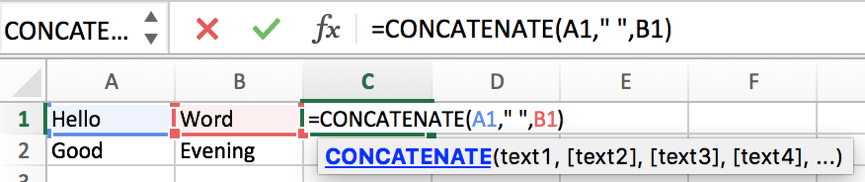Step 2: Press Enter to get the result.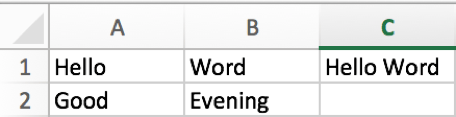Step 3: Copy cell C1 to C2. Words are merged into one cell properly.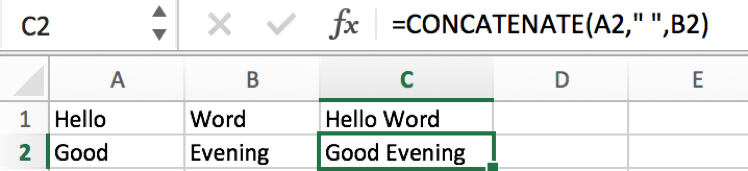b. Insert point “.” between words by CONCATENATE FUNCTION.

A point can be used for concatenate the first name and the last name which are listed in different cells.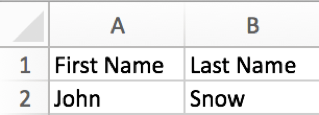We can repeat above steps to concatenate the first name and the last name, but as in this case, we want concatenate names by “.”, so we need to update the formula.

Enter =CONCATENATE(A2,”.”,B2). You can find that we replace “ ” with “.” in the formula.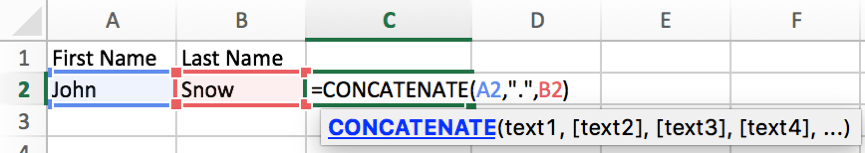Now full name is displayed in C2 properly.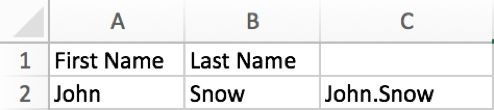c. Insert dash “-” between words by CONCATENATE FUNCTION.

This separator is often used to connect date as a period. See below example.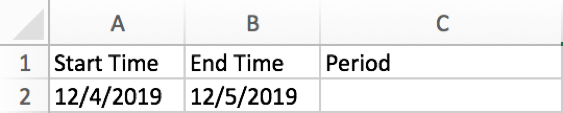Enter =CONCATENATE(A2,”-“,B2). Now you may know that we can enter any separator we want into the quotation marks to connect words.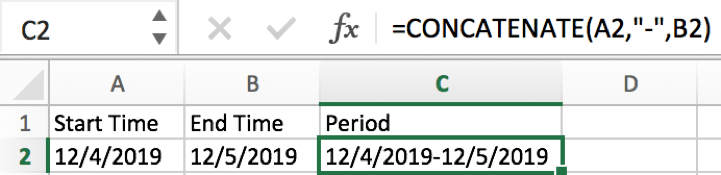Notes: we have to change the format to Text for date, otherwise the concatenate period may be displayed improperly.

## Concatenate words by & symbol

You can also use a & symbol to do connection if you forget to use CONCATENATE function in Excel. Here is a simple way to connect words.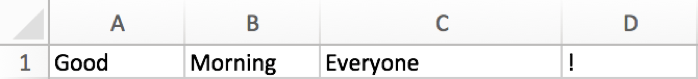In E1, enter =A1&” “&B1&” “&C1&” “&D1. There is a space between double quotes. That’s because we want to use a space to separate each word.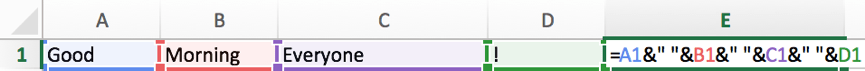A complete sentence is displayed in E1.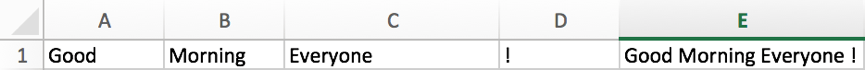Related Posts

If Cell is This Value or That Value

IF function is frequently used in Excel worksheet to return you expect “true value” or “false value” based on the result of logical test. If you want to see if a cell is A or B, and if one of ...

If Value is Greater Than A Certain Value

IF function is frequently used in Excel worksheet to return you expect “true value” or “false value” based on the logical test result. If you want to see if a value in one cell is greater than a specific value, ...

If Cell is Not Blank

IF function is frequently used in Excel worksheet to return you expect “true value” or “false value” based on the result of created logical test. If you want to see if a cell is blank or not, and leave some ...

VBA Macro For VLOOKUP From Another Sheet

In the previous post, you should know that how to fix or remove the #N/A error when using VLOOKUP formula to lookup value from another sheet. And this post will show you how to use VBA code to vlookup data ...

If Cell is Blank

IF function is frequently used in Excel worksheet to return you expect “true value” or “false value” based on the result of created logical test. If you want to see if a cell is blank or not, and leave some ...

If Cell Equals Certain Text String

IF function is frequently used in Excel worksheet to return you expect “true value” or “false value” based on the result of created logical test. If you want to see if cell equals a certain text string like “Win”, you ...

If Cell Contains Either Text1 or Text2

IF function is frequently used in Excel worksheet to return “true value” or “false value” based on the logical test result. If you want to see if cell contains certain substring1 like “abc” or substring2 like “def”, and returns true ...

If Cell Contains Certain Text OR Equals Certain Text

IF cell equals certain text IF function is frequently used in Excel worksheet to return “true value” or “false value” based on the logical test result. If you want to test values to see if they equal certain text like ...

VLOOKUP From Another Sheet Not Working

In the previous post, you should know that how to fix or remove the #N/A error when using VLOOKUP formula to lookup value from another sheet. And this post will show you reasons why your VLOOKUP formula is not working ...

If Cell Begins with One of Three Supplied Characters

If you want to test values to see if they begin with some given specific characters like “x”, ”y”, or “z”, you can create a formula with COUNTIF and SUM functions to return results. EXAMPLE You can see “TRUE” or ...

Sidebar